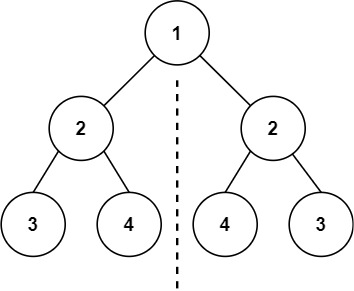# Leetcode 101/100 - Symmetric tree / Same tree

Note

• Intuitive way is to use recursion.
• There are three situations
• Both are null, which means left subtree and right subtree are the same.
• One of them is null, so it’s false
• Both are not null, then we need recursion, comapre val first then use &&. Be careful that we need to compare left.left, right.right and left.right, right.left.

Given the root of a binary tree, check whether it is a mirror of itself (i.e., symmetric around its center).

Example